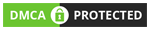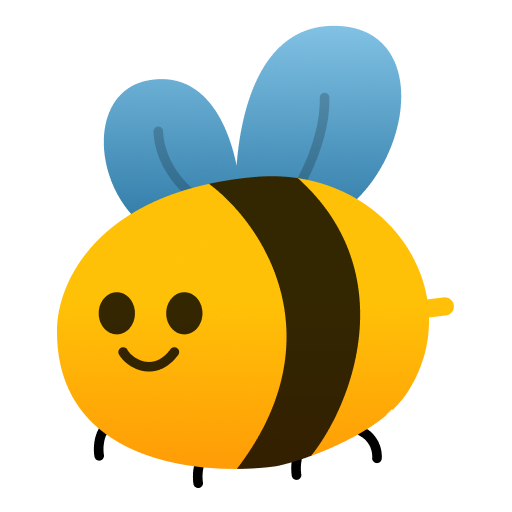Computer Science Online Courses

Android MCQs

C Programming Frequently Asked Questions Multiple Choice Questions (MCQ), Android quiz answers PDF to study android online course for android classes. if statement Multiple Choice Questions and Answers (MCQs), Android quiz questions for online degrees. "C Programming Frequently Asked Questions" PDF Book: learning c language with interactive questions and answers, introduction to computer questions and answers, c programming practice exercises with answers test prep for information and communication technology.

"By evaluating the following statement where x=25.0 and y=15.0 <code>if (y < 15.0 && y >= 0.0)<br>x = 5 * y;<br>else<br>x = 2 * y; </code>, the value of x is" MCQ PDF: c programming frequently asked questions with choices 30, 45, 60, and 75 for online degrees. Study c programming frequently asked questions quiz questions for merit scholarship test and certificate programs for associates in computer science.

## MCQs on C Programming Frequently Asked Questions Quiz

MCQ: By evaluating the following statement where x=25.0 and y=15.0 `if (y < 15.0 && y >= 0.0)x = 5 * y;elsex = 2 * y; `, the value of x is

30
45
60
75

MCQ: By evaluating the following statement where x=25.0 and y=15.0`if (y < 15.0){ if (y >= 0.0) x = 5 * y; else x = 2 * y;}else x = 3 * y;` , the value of x is

15
30
45
75

MCQ: By evaluating the following statement ` if (12 < 12 ) printf("less");elseprintf("not less")};` , the output is

less
not less
LESS
NOT LESS

MCQ: By evaluating the following statement where var1=25.12 and var2=15.00 `if (var1 <= var2)printf("less or equal/n");elseprintf("Greater then\n")};` , the output is

less or equal
Greater then
less or equal Greater then
Compiler error

MCQ: By evaluating the following statement where x=25.0 and y=15.0 `if (y!= (x-10.0))x = x - 10.0;elsex = x / 2.0;`, the value of x is

25
12
15
12.5

### More Topics from Android Course```General Home Site Map Privacy Policy Affiliate Disclosure Contact Us Social Links Facebook Twitter YouTube Mobile Apps Android iOSAs an Amazon Associate I earn from qualifying purchases. MCQsLearn Web Portal Copyright © 2014-2022. All rights reserved.Try our FREE mobile apps for an amazing & interactive experience. Buy PREMIUM with trial period to see all analytics, complete history, answer keys to improve your premium insights. We assure you to enjoy PREMIUM! function checkAttempt(element, number) { var answer = document.getElementById("answer-" + number).innerHTML; var options = Array.from(document.getElementsByClassName("option-" + number)); if (answer == element.id.split("-")) { element.classList.add("correct-option"); options.forEach(function(option) { option.classList.add("disabled"); }); } else { element.classList.add("wrong-option"); options.forEach(function(option) { option.classList.add("disabled"); if (answer == option.id.split("-")) { \$("." + option.classList).delay(500).queue(function() { this.classList.add("correct-option"); }); } }); } } const validPage = window.location.href != "https://mcqslearn.com/apps/free-apple-android-apps-to-download.php"; function getCookie(cname) { let name = cname + "="; let decodedCookie = decodeURIComponent(document.cookie); let ca = decodedCookie.split(';'); for (let i = 0; i < ca.length; i++) { let c = ca[i]; while (c.charAt(0) == ' ') { c = c.substring(1); } if (c.indexOf(name) == 0) { return c.substring(name.length, c.length); } } return ""; } const _modal = document.getElementById("resetCourseModal"); const modal = new bootstrap.Modal(_modal); setTimeout(function() { if (!getCookie("initModal") && validPage) { modal.show(); const exp = new Date(); exp.setTime(exp.getTime() + (12 * 60 * 60 * 1000)); const utcExp = exp.toUTCString(); document.cookie = "initModal=true;expires=" + utcExp + ";path=/"; } }, 5000); document.body.addEventListener("mouseleave", function() { if (!getCookie("exitModal") && validPage) { modal.show(); const exp = new Date(); exp.setTime(exp.getTime() + (12 * 60 * 60 * 1000)); const utcExp = exp.toUTCString(); document.cookie = "exitModal=true;expires=" + utcExp + ";path=/"; } }); ```